Mobile QR CodePortable microwave generator, atmospheric-pressure plasma, low-temperature plasma, power efficiency, impedance matching

## I. INTRODUCTION

Plasma is the fourth state of matter; it is a highly reactive mixture of ions, free radicals and electrons (1). It can be generated by using a strong electromagnetic field or heat to excite neutral gas (2) and has been applied in various fields (3-6). Fig. 1 shows the plasma treatment applications in soft biomedical areas such as sterilization (7), coagulation (8), and wound healing (9). These applications require portable plasma generators that operate at atmospheric pressure and room temperature (10-13). Atmospheric pressure plasma (APP) can be generated without requiring a separate chamber, and is therefore suitable for use in low-cost, portable devices (14).

Fig. 1. Microwave-excited plasma to biomedical applications.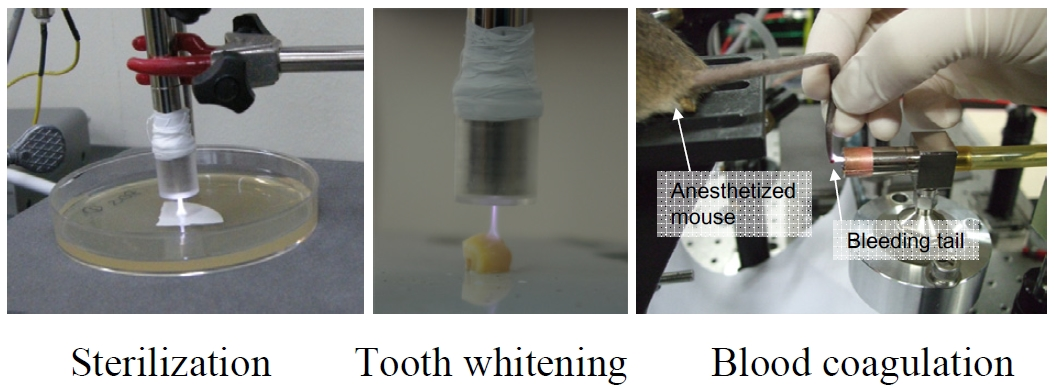The characteristics of the APP depend on the excitation frequency of the power source (15). Microwave-excited APP (${\mu}$-APP) is ignited by a microwave-frequency signal, and has the great advantage of selectively transferring energy to electrons, which are less massive than ions or neutrons (16). Therefore, ${\mu}$-APP produces numerous energetic electrons, which generate abundant reactive species. ${\mu}$-APP is suitable for portable devices because it ignites at low power (17-20).

The ${\lambda}$/4 coaxial transmission line resonator (CTLR) is the most promising ${\mu}$-APP candidate, because it can be implemented in a small pen-type portable device (21). In Fig. 2, the ${\lambda}$/4 CTLR receives microwave power through a 50-Ω cable and generates the ${\mu}$-APP at the open end of a plasma gun. The CTLR has a transmission line structure that consists of an inner electrode and an outer electrode. The transmission lines in the open-end and short-end directions act as a capacitor and an inductor, respectively, so the resonator performs narrow-band peaking. Therefore, the intensity of microwave becomes maximum at the open end and minimum at the short end, and as a result create a strong electric field at the open end. However, after the plasma ignites, the plasma bulk with sheath layer changes the circuit model from an open circuit to a series connection of a capacitor and a resistor as shown in Fig. 3. Fig. 4 shows a shift of resonance frequency by the plasma ignition. The shift of the resonance frequency causes an increase in the power needed to sustain the plasma after ignition. Also, sustaining the plasma under mismatched impedance can cause a large power reflection, which can reduce the lifetime of the power module.

Fig. 3. Equivalent circuit models of the CTLR before and after plasma ignition.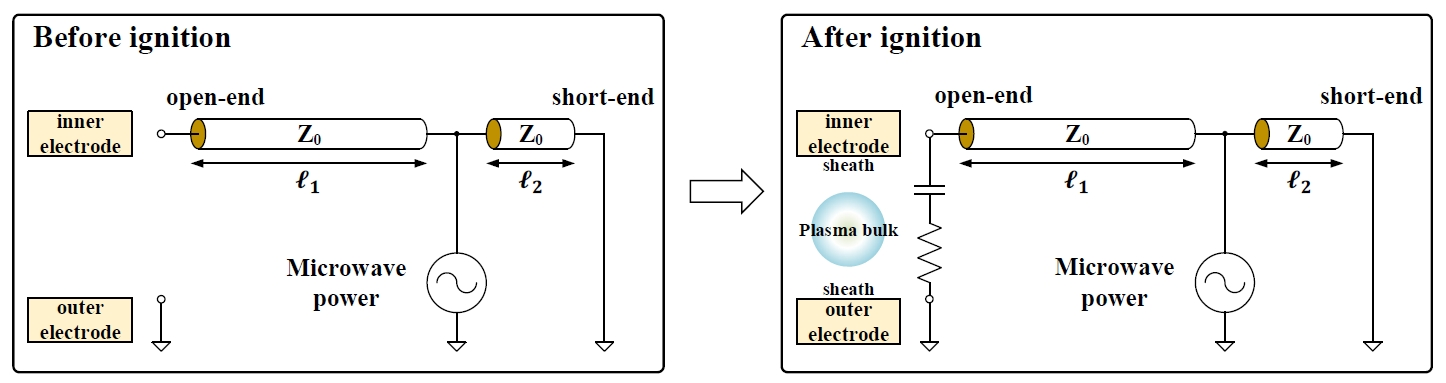An adaptive resonance frequency tracking loop has been developed (22) to overcome the failure of the impedance matching caused by the plasma ignition. A palm-sized microwave power module continuously tracks the shift of resonance frequency and adaptively changes the frequency of the microwave feed. This frequency tracking scheme greatly reduces the waste of power. In addition, a power regulation loop that generates stable microwave power regardless of system temperature changes was implemented.

This paper presents a portable ${\mu}$-APP system with a single-chip microwave-excited plasma driver IC, which includes a resonance-frequency tracking scheme and an output-power regulation scheme. This work proposes a single-chip CMOS controller IC for microwave plasma generation. The IC includes a driver amplifier which provides an input power to an external commercial power amplifier for actual plasma generation. The CTLR is designed to have a resonance frequency in the 2.4-GHz band. The combined ${\mu}$-APP system includes a loop that automatically tracks the maximum power efficiency, and thereby achieves a sufficiently small portable device and a high power efficiency.

## II. PROPOSED MICROWAVE-EXCITED PLASMA DRIVER IC

Fig. 5 shows the proposed IC which consists of three main blocks: a frequency-control block (FCB), a power-regulation block (PRB), and a power-detection block (PDB). The FCB observes the reflected power that is digitized by the PDB and changes the microwave frequency to reduce the magnitude of the reflected power. The PRB monitors the power supplied to the CTLR and adjusts the output level of the power amplifier so that a constant plasma jet is generated. The PDB samples the forward and reflected power from the input of the CTLR, converts them to digital data and transfers them to the FCB and PRB. Because both the frequency control loop and the power regulation loop are involved in the power delivered to the CTLR, the bandwidths of the two loops should be carefully designed to ensure system stability. In this work, the frequency control loop is updated once in every 32 updates of the power regulation loop. An on-chip regulator receives the 3.3-V voltage and generates a low-noise 1.8-V supply voltage for all blocks except front-end circuits. The relaxation oscillator in the IC provides low-speed (< 1 MHz) clocks (CLK$_{1}$, CLK$_{2}$, and CLK$_{\mathrm{ADC}}$) for operation of digital blocks and ADC. Register banks are controlled by an I$^{2}$C interface block.

Fig. 5. Block diagram of the proposed microwave-excited plasma driver IC.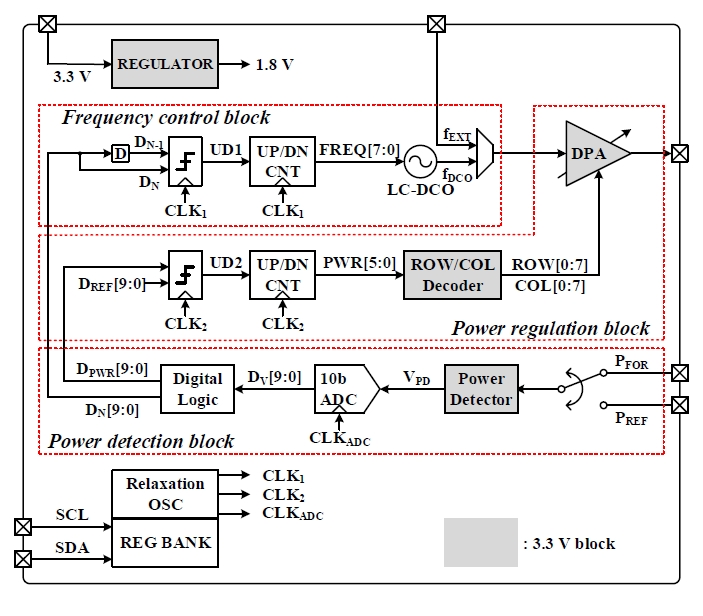### 1. Frequency Control Block

The FCB uses an LC-based digitally controlled oscillator (LC-DCO) to generate a 2.4-GHz band microwave signal. Fig. 6 shows the circuit schematic and output frequency range of the 8-bit LC-DCO. The resonant tank in the DCO consists of an on-chip spiral inductor and a variable capacitor that takes 8-bit digital input. The LC resonance frequency can be tuned by controlling the 8-bit digital input $\textit{FREQ[7:0]}$. In order for the FCB to stably lock to the resonance frequency of CTLR, a 2.4 GHz band LC-DCO was designed to have a tunable frequency range of 80 MHz with an 8-bit code. Additional band selection capacitors are also added to cope with nonzero frequency shifts caused by plasma ignition. The overall frequency range with additional band selection capacitors is 2.28 to 2.53 GHz. The generated microwave signal $\textit{f}$$_{DCO} is applied to the input of the power amplifier through a microwave selection multiplexer (MUX). Fig. 6. (a) Circuit schematic of the 8-bit LC-DCO, (b) Output frequency range of the LC-DCO.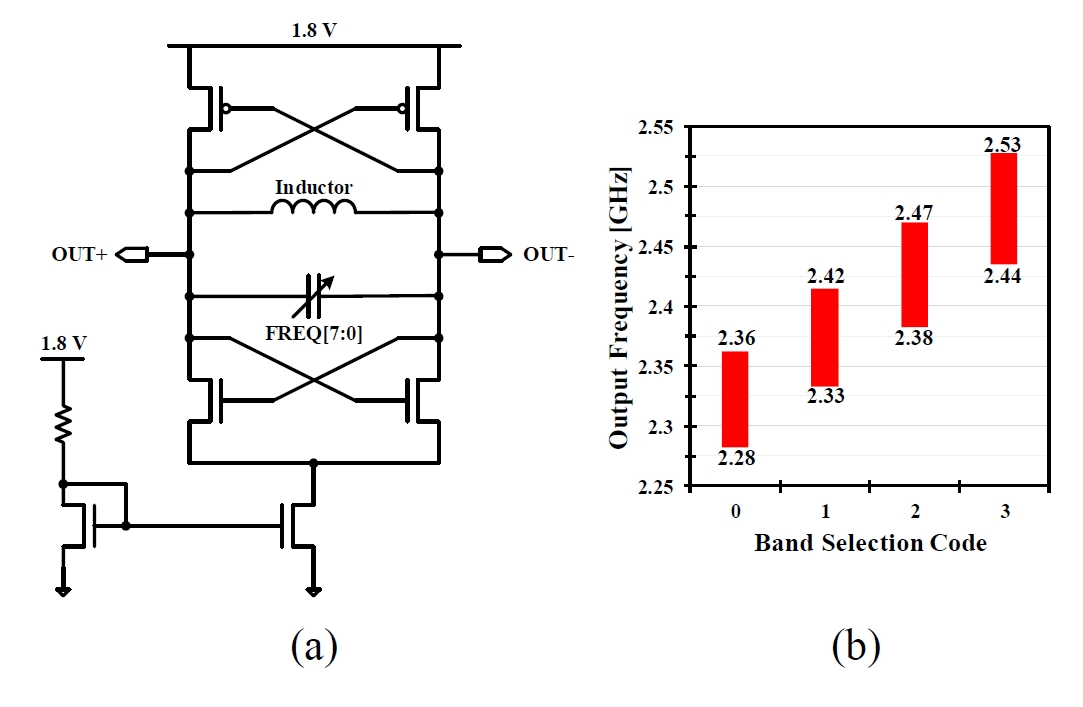A digital comparator in the FCB compares the digitized reflected power \textit{D}$$_{N}$ at the current frequency with the digitized reflected power $\textit{D}$$_{N-1} at the previous frequency. If \textit{D}$$_{N}$ ${\geq}$ $\textit{D}$$_{N-1}, the comparator output \textit{UD1} = +1; if \textit{D}$$_{N}$ < $\textit{D}$$_{N-1}, then \textit{UD1} = -1. The comparator output is applied as an input to an UP/DOWN counter to increase or decrease \textit{FREQ[7:0]} by 1. The changed counter value is applied to the 8-bit variable capacitor of the LC-DCO so the output frequency is changed by (80/255) MHz. The FCB receives the new reflected power at the changed frequency and repeats the comparison process. An UP/DOWN counter acts as an integrating filter to provide the single dominant pole. Therefore, the loop of the FCB is a first-order system and unconditionally stable. If the change in frequency causes an increase in the reflected power, the output of the comparator has the opposite sign as the previous output. As a result, the output frequency changes in the direction opposite to the previous change and moves toward to the minimum point of the reflection coefficient (\textit{S}$$_{11}$). In the opposite case, the comparator outputs a value with the same sign, so the output frequency changes in the same direction as the previous change, and the minimum point is tracked in the same way. This simple gradient-search algorithm, makes the 8-bit LC-DCO input converge to the optimal code that minimizes the reflected power.

### 2. Power Regulation Block

The main function of the PRB is to provide a constant output power to the CTLR regardless of variation in the power supply or the temperature of the device. In Fig. 7, the microwave signal generated by the FCB is applied to the input of the digitally power-controllable amplifier (DPA). The microwave selection MUX determines whether to use of $\textit{f}$$_{DCO} or an external microwave signal \textit{f}$$_{EXT}$, then applies the chosen microwave signal to the inverter buffer. The buffer amplifies the signal and adjusts the DC voltage level with an AC-coupling capacitor and resistors. The adjusted signal is applied to the input of a DPA that consists of 64 cascode cells. The upper NMOS gate driven is by logic gates, and determines the whether a single cascode cell is turned on or off. $\textit{ROW[0:7]}$ and $\textit{COL[0:7]}$ control the output power of the DPA by determining the number of cells that are turned on. The output terminals of the DPA bear is a choke inductor to supply DC current to the DPA and passive elements for 50-Ω matching. In Fig. 8, the measured output power of the DPA increases with increase in the number of DPA cells that are turned on. The measured maximum output power of the DPA is 19.38 dBm and the minimum output power is -20.73 dBm.

Fig. 7. Circuit schematic of the digitally power-controllable amplifier.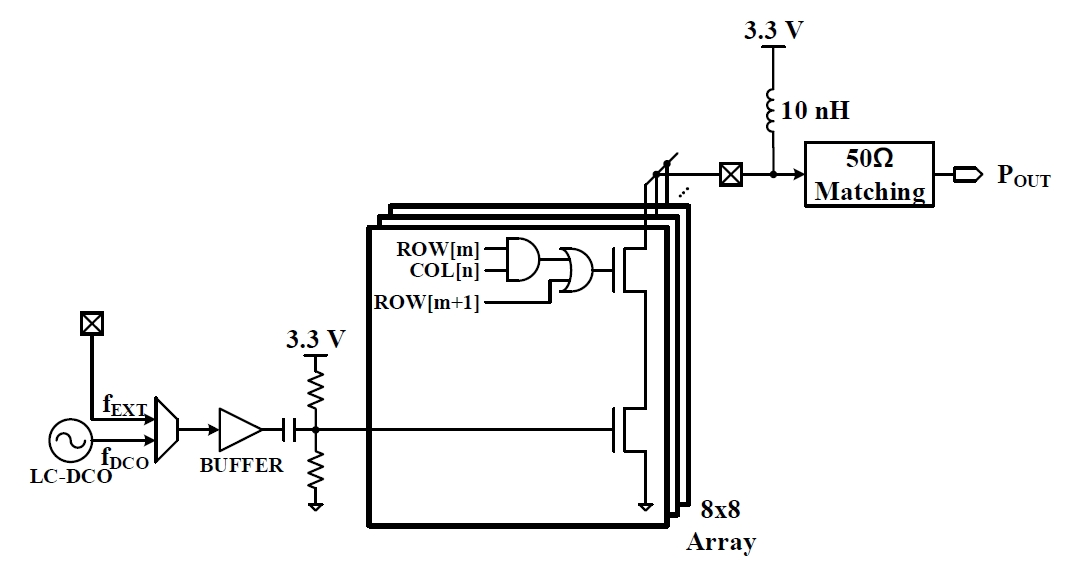Fig. 8. Measured output power ($\textit{P}$$_{out}) as a function of 6-bit input code.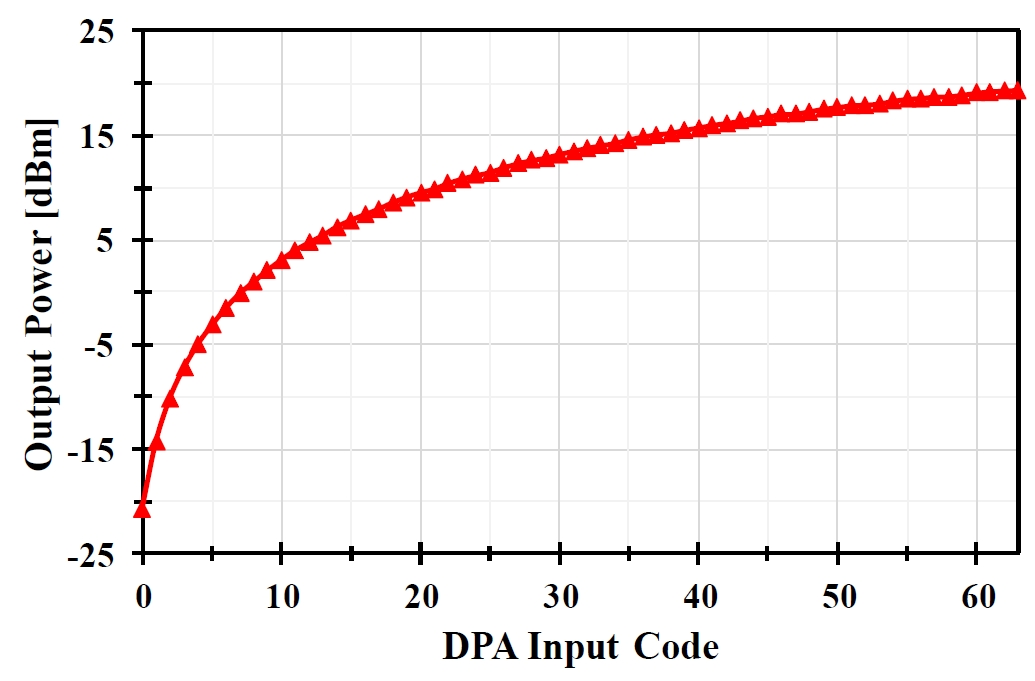The power regulation loop of the PRB operates such that the effective power \textit{P}$$_{eff}$ (i.e., forward power minus reflected power), is equal to the target output power. The digitized effective power $\textit{D}$$_{PWR} is received from the digital logic block of the PDB. A digital comparator in the PRB compares \textit{D}$$_{PWR}$ with the digitized target power $\textit{D}$$_{REF} value and outputs +1 or -1 (\textit{UD2}). An UP/DOWN counter in the PRB changes the counter value \textit{PWR[5:0]} according to the \textit{UD2} value, then \textit{PWR[5:0]} is converted to \textit{ROW[0:7]} and \textit{COL[0:7]} values by a ROW/COL decoder. While the loop is active, the plasma output of the CTLR is regulated. ### 3. Power Detection Block Operation of the FCB and PRB requires the reflected power and effective power of the CTLR. The PDB receives forward and reflected signals as input, then transfers the digitized reflected power to the FCB and the digitized \textit{P}$$_{eff}$ to the PRB. The power detector shown in Fig. 9 is the front-end circuit of the PRB. Two directional couplers outside the IC sample the signal at the CTLR: one samples the forward power $\textit{P}$$_{FOR} and one samples the reflected power \textit{P}$$_{REF}$; then the couplers apply these powers to the power detector. The input ports of the power detector are matched to 50 Ω; AC coupling capacitors and resistors adjust the DC voltage level. As shown in Fig. 10, the measured output voltage of the power detector increases exponentially with increase in the input power at 2.4 GHz.

Fig. 10. Measured output voltage ($V_{PD}$) as a function of input power.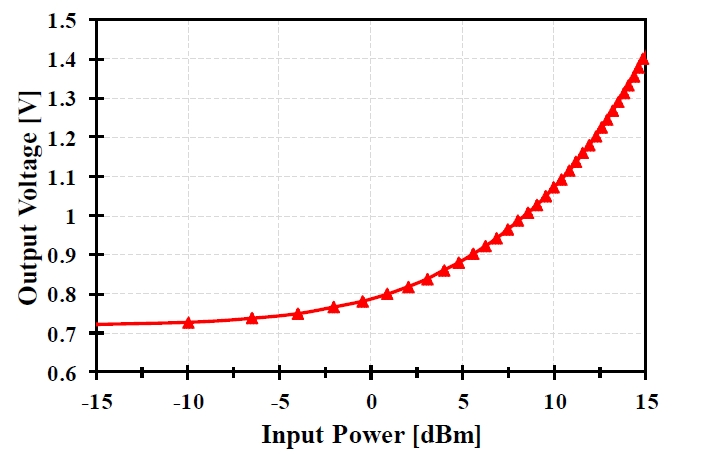The output voltage $\textit{V}$$_{PD} of the power detector is converted to a 10-bit digital value (\textit{D}$$_{V}$$\textit{[9:0]}) by an analog-to-digital converter (ADC) to reduce sensitivity to noise. Fig. 11 and 12 illustrate the block diagram and timing diagram of the implemented 10-bit ADC. For energy efficiency, the proposed system uses successive approximation architecture (SAR). An asynchronous dynamic logic block (23) in the ADC receives the ADC clock from the relaxation oscillator and generates timing logics of internal blocks such as capacitor DAC and comparator. After analog input is converted, the ADC automatically enters standby state, so the total power consumption of the ADC is minimized. Fig. 13 shows simulated power detector output and corresponding ADC output when frequency changes. When the frequency is at the optimum (2.34 GHz), the power detector output voltage changes only with 0.3 mV/MHz, requiring higher resolution of ADC. To achieve a lock at the optimum within {\pm}5-MHz deviation, the required ADC resolution is 10-bit assuming that ADC input range is 1.8 V. The sampling rate of ADC is designed to be not more than 1 MHz which should be significantly smaller than the loop bandwidth of the frequency tracking through the external power amplifier, the CTLR and the IC. The synthesized digital logic block compares digitized forward and reflected power to compute the reflection coefficient and \textit{P}$$_{eff}$, and transfers it to the FCB and PRB.

Fig. 11. Block diagram of the 10-bit asynchronous SAR ADC.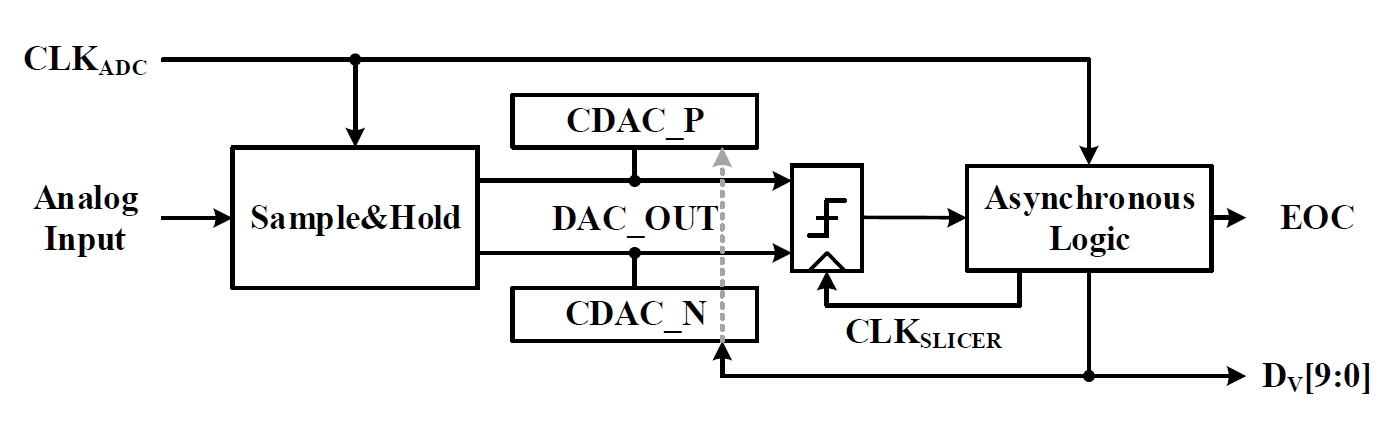Fig. 13. $\textit{P}$$_{REF} measurement results of power detector and 10-bit ADC.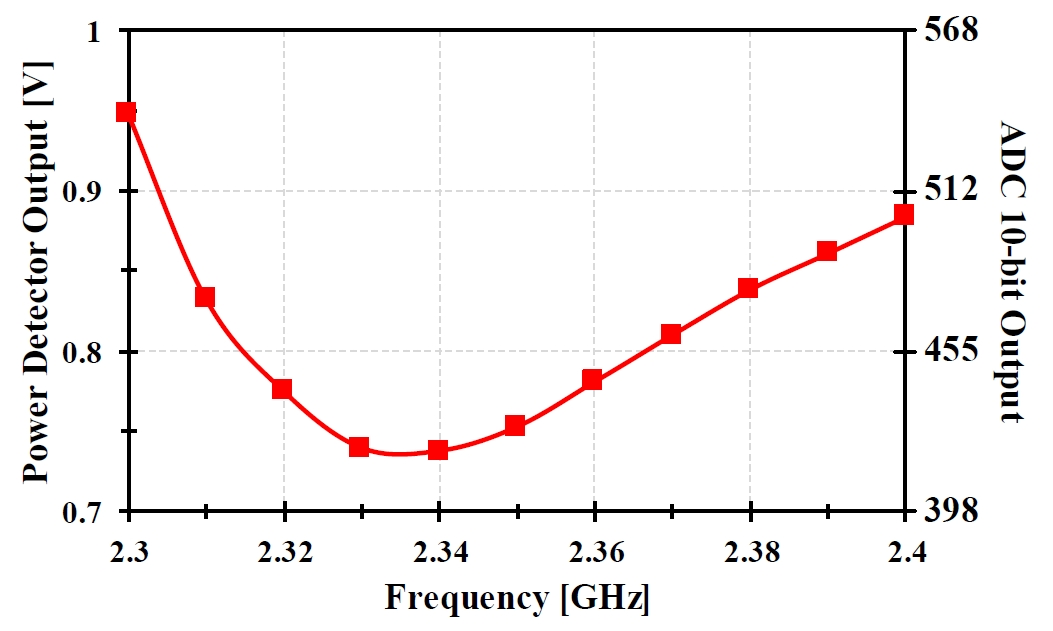## III. 2.4-GHZ COAXIAL TRANSMISSION LINE RESONATOR The CTLR (21) in the proposed system is designed based on the principle of a quarter-wave resonator. The designed CTLR shown in Fig. 14 consists of an inner electrode and an outer electrode. The outer electrode is connected to the ground, and the inner electrode is connected to the microwave power so that an electric field forms between them. The generated electric field excites Argon/Helium gas that is fed into a small hole of the short-end; the result is a plasma jet. An equivalent model of the CTLR structure is shown in Fig. 15. The input impedance at the power-feeding port is the parallel sum of impedance \textit{Z}$$_{L1}$ and impedance $\textit{Z}$$_{L2}. When the plasma is not generated, the impedance at the open end is infinite (\textit{Z}$$_{P}$ =${\infty}$),

##### (1)
$$Z_{L 1}=Z_{o} \frac{1}{\tanh \left(j k \ell_{1}\right)}$$

and

##### (2)
$$Z_{L 2}=Z_{o} \tan \mathrm{h}\left(j k \ell_{2}\right),$$

where Zo is the characteristic impedance of the coaxial transmission line (50 Ω), j=${\sqrt{}}$(-1), $l_{1}$ is the length of the transmission line from power-feeding port to the open end, $l_{2}$ is the length of the transmission line from power-feeding port to short-end, $k={\beta}-{j \alpha}$ is the propagation constant, ${\alpha}$ is the attenuation constant, and ${\beta}={\lambda}/4$ is the phase constant of the coaxial transmission line. $l_{1}$ and $l_{2}$ of the coaxial transmission lines are short, so the impedance $\textit{Z}_{L1}$ and $\textit{Z}_{L2}$ can be assumed to be purely capacitive and purely inductive respectively, so LC resonance results. The input impedance seen at the microwave power-feeding port is

##### (3)
$$\begin{array}{c} Z_{\text {in}}=Z_{L 1} \| Z_{L 2} \approx Z_{o}\left[\left(\frac{\alpha \ell_{1}}{\sin ^{2}\left(\beta \ell_{1}\right)}+\frac{\alpha \ell_{2}}{\cos ^{2}\left(\beta \ell_{2}\right)}\right)\right. \\ \left.+j\left(\frac{-1}{\tan \left(\beta \ell_{1}\right)}+\tan \left(\beta \ell_{2}\right)\right)\right]^{-1} \end{array},$$

and by making the total length $l_{1}$ + $l_{2}$ = ${\lambda}$/4, the imaginary part of the input impedance disappears without any condition. The real part of the input impedance can be adjusted by locating the power-feeding point at the spot where $\textit{Z}$$_{in} matches \textit{Z}$$_{o}$.

##### (5)
$$S_{11}[d B]=20 \log _{10}\left(\frac{Z_{i n}-Z_{o}}{Z_{i n}+Z_{o}}\right)$$

where $\textit{Z}$ $_{p}$ = $\textit{R}$$_{p} - j\textit{X}$$_{p}$, $\textit{R}$$_{p} is resistive impedance of the plasma jet and \textit{X}$$_{p}$ is reactive impedance of the plasma jet. By fitting the theoretically calculated reflection coefficient and the measured reflection coefficient, resistance and reactance can be estimated. In Fig. 20, CTLR reflection coefficients were measured in the frequency range from 2.3 to 2.4 GHz at forward powers of 1.0, 1.5, and 2.0 W. The reflection coefficients of the CTLR were measured using forward and reflected power measured by two directional couplers. As the power of the plasma jet increased from 1.0 W to 2.0 W, the estimated plasma impedance decreased and the reflection characteristics of the CTLR degraded. The measured plasma impedance of the plasma jet is shown in Fig. 21.

Fig. 20. Measurement result of $\textit{S}$$_{11} before plasma ignition and after plasma ignition with forward input power variation.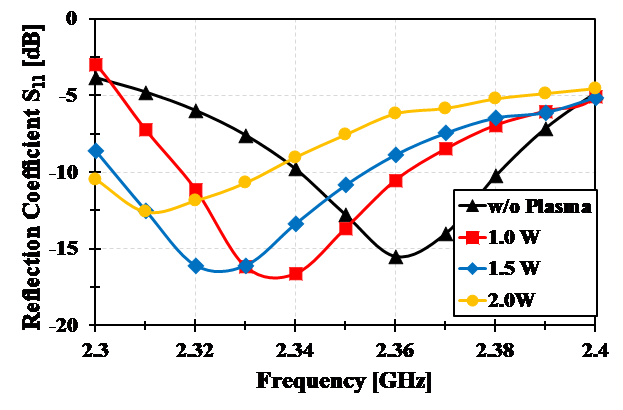Fig. 21. Measured resistance and reactance of the plasma jet with forward input power variation.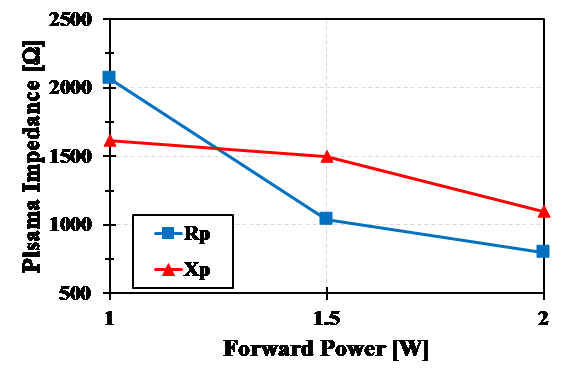### 2. Frequency Tracking The plasma jet impedance \textit{Z}$$_{p}$ decreased as the forward power of the resonator increased, so that the minimum point of $\textit{S}$$_{11} shifted to a lower frequency. When the frequency tracking mode was activated, the minimum point of \textit{S}$$_{11}$ is searched regardless of the initial position of the frequency. Fig. 22 shows the measured DCO frequency codes before and after the plasma ignition while the frequency tracking mode was turned on. The DCO code converged to the optimal frequency code, whether the initial code was far from or near to the optimal code. After the plasma ignition, the DCO frequency code converged to the shifted optimum frequency code after the frequency-tracking operation was iterated. After the convergence, the DCO code had a ripple in the range of ${\pm}$10 from the optimal code, so the locking range of the microwave frequency was 6.5 MHz; this error is caused by power detector gain and ADC resolution limitations; it slightly reduced power efficiency but the overall power efficiency was greatly increased.

Fig. 22. Measured DCO frequency codes while frequency tracking mode is on (Dashed lines represent the optimal DCO frequency codes).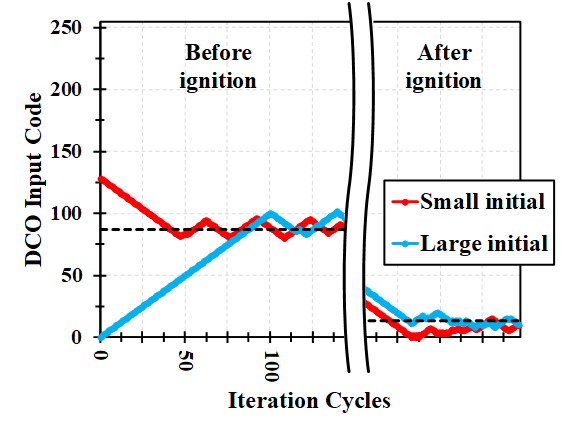### 3. Power-transfer Efficiency

The power-transfer efficiency ($\textit{PT}_{eff}$), which can be calculated as

##### (6)
$$P T_{e f f}=\frac{P_{F O R}}{P_{F O R}+P_{R E F}} \times 100$$

where $\textit{P}$$_{FOR} is forward power in the CTLR and \textit{P}$$_{REF}$ is reflected power in the CTLR. Fig. 23 compares the $\textit{PT}_{eff}$ for two cases of frequency control: 1) frequency fixed at the initial resonance frequency of 2.36 GHz without frequency tracking, and 2) frequency adaptively tuned with the minimum point of $\textit{S}$$_{11}$ tracking loop. In case 1), the power-transfer efficiency decreased significantly as the forward input power increased. The 95.2 % power-transfer efficiency before plasma ignition decreased to 72.1 % when the forward input power was 2.0 W. In case 2), the optimal frequency was tracked according to the change of the input impedance of the CTLR, so the power-transfer efficiency was similar to the initial efficiency of 95.1 %. The measured power-transfer efficiency was 94.8, 94.4, and 92.5 % at forward power of 1.0, 1.5, and 2.0 W, respectively. The measured performance of the implemented IC is summarized in Table 1.

Table 1. Performance summary of implemented chip

 Process 0.18 μm CMOS Output power -20.7 dBm ~ 19.4 dBm Frequency range 2.28 GHz ~ 2.53 GHz Power-transfer efficiency 94.8 % @ 1.0 W output 94.4 % @ 1.5 W output 92.5 % @ 2.0 W output Power consumption 127 mW @ 3.3 V Chip area 2.1 mm × 1.1 mm

Fig. 23. Power-transfer efficiency from power module to CTLR with fixed frequency and frequency tracking.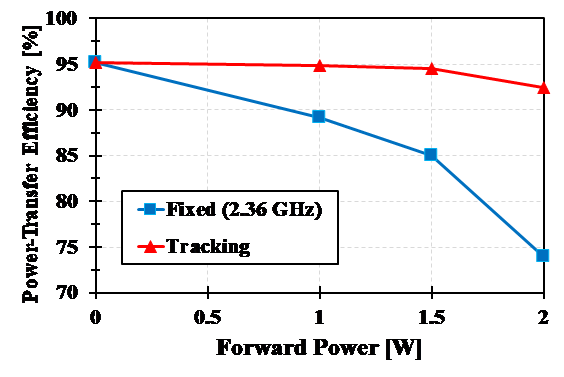## V. CONCLUSION

This paper presents a portable ${\mu}$-APP system that uses a single-chip microwave plasma driver IC that has two feedback loops: (1) a resonance-frequency-tracking loop that tracks the shift of the minimum reflection frequency caused by changes in the plasma impedance, and (2) an output-power-regulation loop that keeps the intensity of the plasma jet constant. The resonance frequency tracking scheme finds the minimum reflection frequency by continuously running a simplified gradient search. The output power regulation scheme finds a DPA code so that the effective power level would be a given constant. The power module including the implemented IC and a power amplification block had a maximum output power of 4.0 W and successfully tracked the shifted optimum frequency after the plasma formation. The power efficiency of the system with the frequency tracking scheme was measured at 92.8 %, which is much higher than the 72.6 % efficiency that is achieved when the system is operated at a fixed frequency. The output power regulation scheme was applied to provide a constant effective power within ${\pm}$ 5 % ripple error. The proposed system is suited for portable low-power biomedical microwave plasma devices.

### ACKNOWLEDGMENTS

This research was supported by the Bio & Medical Technology Development Program of the National Research Foundation (NRF) funded by the Korean government (MSIT) (No. NRF-2015M3A9E2066861).

### REFERENCES

1
Goldston R. J., Rutherford P. H., 1995, Introduction to Plasma Physics, London, U.K.: IOP, pp. 1-22
Conards H., Schmidt M., Nov 2000, Plasma generation and plasma sources, Plasma Sources Sci. Technol., Vol. 9, No. 4, pp. 441-4543
Laroussi M., Jun 2009, Low-temperature plasmas for medicine?, IEEE Trans. Plasma Sci., Vol. 37, No. 6, pp. 714-7254
Bai B., Li X., Xu J., Liu Y., Aug 2015, Reflections of electromagnetic waves obliquely incident on a multilayer stealth structure with plasma and radar absorbing material, IEEE Trans. Plasma Sci., Vol. 43, No. 8, pp. 2588-25975
Park S.-J., Aug 2010, Inactivation of S. mutants using an atmospheric plasma driven by a palm-size-integrated microwave power module, IEEE Trans.Plasma Sci., Vol. 38, No. 8, pp. 1956-19626
Corke T. C., Enloe C. L., Wilkinson S. P., Sep 2010, Dielectric barrier discharge plasma actuators for flow control, Annu. Rev. Fluid Mech., Vol. 42, pp. 505-5297
Sun P., Aug 2010, Tooth whitening with hydrogen peroxide assisted by a direct-current cold atmospheric-pressure air plasma microjet, IEEE Trans. Plasma Sci., Vol. 38, No. 8, pp. 1892-18968
Choi J., Mohamed A.-A. H., Kang S. K., Woo K. C., Kim K. T., Lee J. K., Mar 2010, 900-MHz nonthermal atmospheric pressure plasma jet for biomedical applications, Plasma Process. Polym., Vol. 7, No. 3-4, pp. 258-2639
Olszewski P., Li J. F., Liu D. X., Walsh J. L., Aug 2014, Optimizing the electrical excitation of an atmospheric pressure plasma advanced oxidation process, J. Hazardous Mater., Vol. 279, pp. 60-6610
Xu N., Cui X., Fang Z., Shi Y., Zhou R., Apr 2018, A two-mode portable atmospheric pressure air plasma jet device for biomedical applications, IEEE Trans. Plasma Sci., Vol. 46, No. 4, pp. 947-95311
Kang S. K., Kim H. Y., Yun G. S., Lee J. K., 2015, Portable microwave air plasma device for wound healing, Plasma Sour. Sci. Technol., Vol. 24, No. 3, pp. 20-3512
Johnson M. J., Boris D. R., Petrova T. B., Walton S. G., Jan 2019, Characterization of a Compact, Low-Cost Atmospheric-Pressure Plasma Jet Driven by a Piezoelectric Transformer, IEEE Trans. Plasma Sci., Vol. 47, No. 1, pp. 434-44413
Kang S. K., Seo Y. S., Lee H. W., Rehman Aman-ur, Kim G. C., Lee J. K., Oct 2011, Slit shaped microwave induced atmospheric pressure plasma based on a parallel plate transmission line resonator, J. Phys. D, Appl. Phys., Vol. 44, No. 42, pp. 43520114
Park J., Henins I., Hermann H. W., Selwyn G. S., Jan 2000, An atmospheric pressure plasma source, Appl. Phys. Lett., Vol. 76, No. 3, pp. 288-29015
Tendero C., Tixier C., Tristant P., Desmaison J., Leprince P., Jan 2006, Atmospheric pressure plasmas: A review, Spectrochim. Acta B, At. Spectrosc., Vol. 61, No. 1, pp. 2-3016
Kwon H. C., Won I. H., Lee J. K., Apr 2012, Electron heating mode transition induced by ultra-high frequency in atmospheric microplasmas for biomedical applications, Appl. Phys. Lett., Vol. 100, No. 18, pp. 183702-1-183702-417
Gesche R., Kühn S., Andrei C., Oct 2008, Plasma ignition in a quarter-wavelength microwave slot resonator, J. Phys. D, Appl. Phys., Vol. 41, No. 19, pp. 19400318
Iza F., Hopwood J. A., Aug 2003, Low-power microwave plasma source based on a microstrip split-ring resonator, IEEE Trans. on Plasma Sci., Vol. 31, No. 4, pp. 782-78719
Xue J., Hopwood J. A., June 2009, Microwave-Frequency Effects on Microplasma, IEEE Trans. Plasma Sci., Vol. 37, No. 6, pp. 816-82220
Iza F., Hopwood J., May 2005, Split-ring resonator microplasma: Microwave model, plasma impedance and power efficiency, Plasma Sources Sci. Technol., Vol. 14, No. 2, pp. 397-40621
Choi J., Iza F., Do H. J., Lee J. K., Cho M. H., May 2009, Microwave-excited atmospheric-pressure microplasmas based on a coaxial transmission line resonator, Plasma Sour. Sci. Technol., Vol. 18, No. 2, pp. 02502922
Kwon S. K., Park S., Won I. H., Ahn C., Sim J., June 2017, Microwave Plasma Generation With Resonance Frequency Tracking and Power Regulation, IEEE Trans. Plasma Sci., Vol. 45, No. 6, pp. 925-93123
Harpe P. J. A., Zhou C., Bi Y., van der Meijs N. P., Wang X., Philips K., Dolmans G., De Groot H., Jul 2011, A 26 μW 8 bit 10 MS/s asynchronous SAR ADC for low energy radios, IEEE J. Solid-State Circuits, Vol. 46, No. 7, pp. 1585-159524
Kwon H. C., Won I. H., Lee J. K., Apr 2012, Electron heating mode transition induced by ultra-high frequency in atmospheric microplasmas for biomedical applications, Appl. Phys. Lett., Vol. 100, No. 18, pp. 183702-1-183702-4## Author

Cheolmin Ahn received the B.S. degree from the School of Computer Science and Electrical Engineering, and the M.S. degree from the Department of Information and Communication Engineering both from the Handong Global University, Pohang, South Korea, in 2012 and 2014, respectively.

He is currently pursuing the Ph.D. degree in electrical engineering with the Pohang University of Science and Technology, Pohang.

Soon Ku Kwon received the B.S. degree form the School of Electronic Engineering, Soongsil University, Seoul, South Korea, in 2012, and the M.S. degree in electrical engineering from the Pohang University of Science and Technology, Pohang, South Korea, in 2014, where he is currently pursuing the Ph.D. degree in electrical engineering.

His current research interests include microwave plasma generator, microwave-excited plasma for biomedical applications, power module for plasma generation, analog integrated circuit design, and RFICs.

Beomjin Park received the B.S. degree from the School of Computer Science and Electrical Engineering, and the M.S. degree from the Department of Electrical Engineering both from the Pohang University of Science and Technology, Pohang, South Korea, in 2014 and 2016, respectively.

He is currently pursuing the Ph.D. degree in electrical engineering with the Pohang University of Science and Technology, Pohang.

Jahyun Koo received the B.S. degree in Electronic and Electrical Engineering from Hongik University, in 2014 and M.S degree in electronic and electrical engineering from Pohang University of Science and Technology (POSTECH), Korea, in 2016.

He is currently pursuing the Ph.D. degree in electronic and electrical engineering from Pohang University of Science and Technology (POSTECH), Korea.

His research interests include relaxation oscillator and sensor interface circuit

Jae-Yoon Sim received the B.S., M.S., and Ph.D. degrees in electronic and electrical engineering from the Pohang University of Science and Technology (POSTECH), Pohang, South Korea, in 1993, 1995, and 1999, respectively.

From 1999 to 2005, he was a Senior Engineer with Samsung Electronics, Suwon, South Korea.

From 2003 to 2005, he was a Post-Doctoral Researcher with the University of Southern California, Los Angeles, CA, USA.

From 2011 to 2012, he was a Visiting Scholar with the University of Michigan, Ann Arbor, MI, USA.

In 2005, he joined POSTECH, where he is currently a Professor.

From 2017 to 2019, he was the Director of the Joint Research Lab, which is nominated by the Korea Institute of Science and Technology.

Since 2019, he has been the Director of the Scalable Quantum Computer Technology Platform Center, which is an engineering research center nominated by the Korea Ministry of Science and Information and Communication Technology (ICT).

His research interests include sensor interface circuits, highspeed serial/parallel links, phase-locked loops (PLLs), data converters, power module for plasma generation, and quantum computing.

Dr. Sim was a co-recipient of the Takuo Sugano Award from ISSCC 2001 and a recipient of the Special Author-Recognition Award from ISSCC 2013.

He has served on the Technical Program Committees for the IEEE International Solid-State Circuits Conference (ISSCC), the Symposium on VLSI Circuits, and Asian Solid State Circuits Conference.

He has been an IEEE Distinguished Lecturer since 2018.